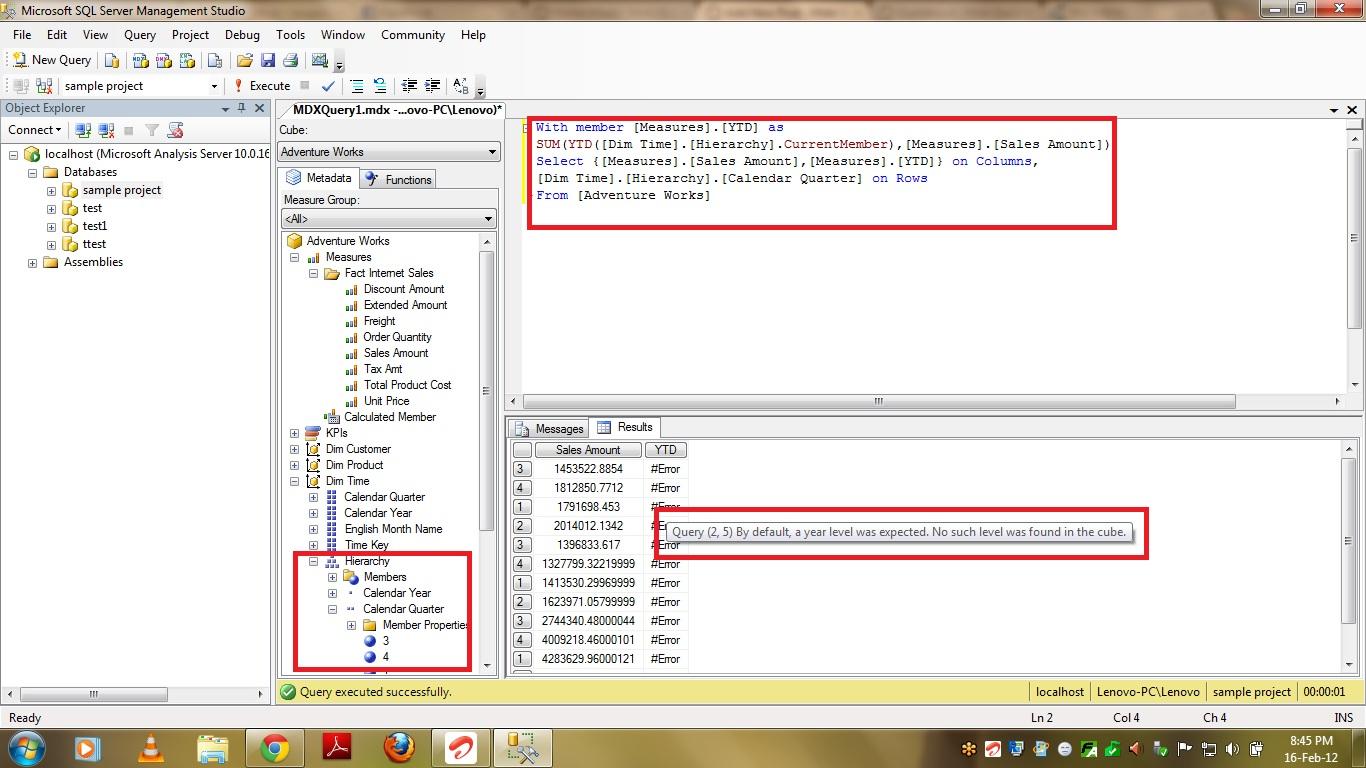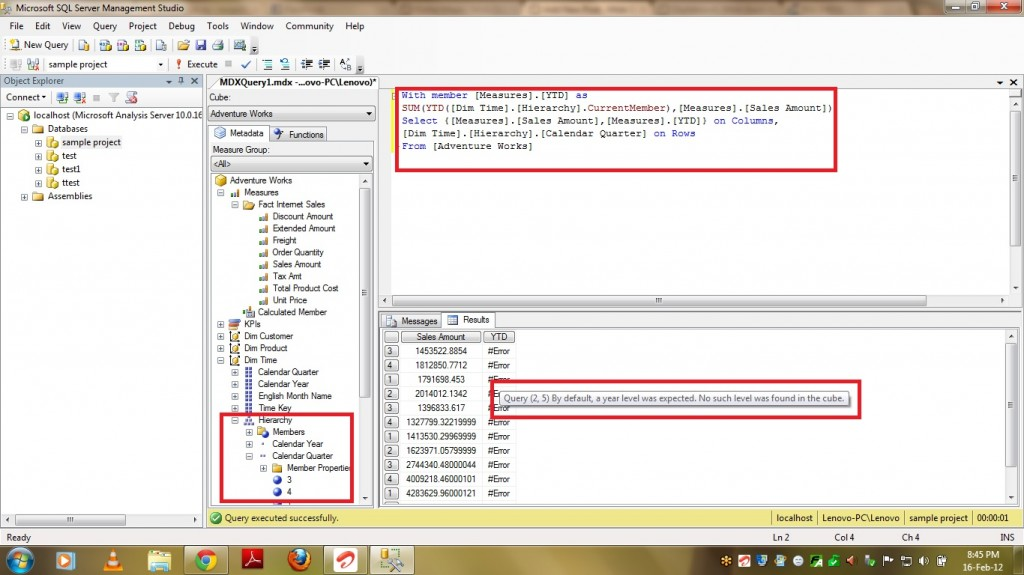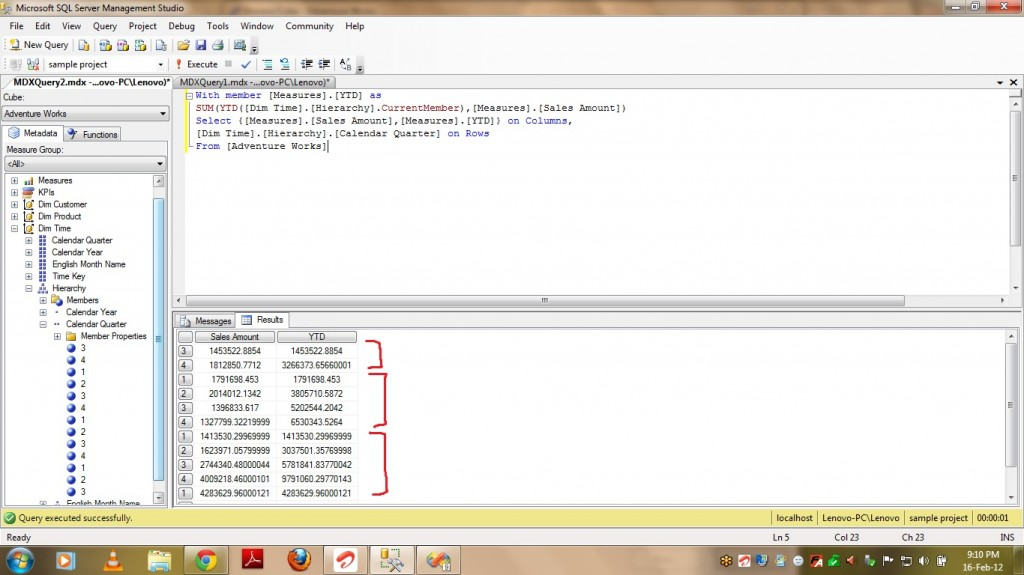# YTD – “By Default, a year level was expected. No such level was found in the cube” Error in MDX

13275Friends,

“By Default, a year level was expected. No such level was found in the cube” is the most common error in MDX when a newbie in MDX is using YTD function. YTD is one of very powerful functions provided my Microsoft in MDX. YTD gives YEAR to DATE value of the measure passed. PFB the syntax of YTD function.

YTD(Member_Exp)

Member_Exp – Valid Member Expression which returns a member from TIME Dimension Hierarchy.

For example if I pass Nov 2011 as a member to the YTD function then it calculates the sum of measure value from Jan 2011 to Nov 2011 and provide you the result. PFB a sample query which shows how to use YTD fucntion.

SUM(YTD(Member_Exp),Measure).

Let’s discuss about the most common error we get when we use YTD function i.e “By Default, a year level was expected. No such level was found in the cube”. When I started my career in SSAS and MDX I tried a lot to solve this issue and make YTD function work. I failed to and used alternate function PERIODSTODATE which helps in achieving the output required. Let’s take a sample query which has YTD function in it.

With member [Measures].[YTD] as
SUM(YTD([Dim Time].[Hierarchy].CurrentMember),[Measures].[Sales Amount])
Select {[Measures].[Sales Amount],[Measures].[YTD]} on Columns,
[Dim Time].[Hierarchy].[Calendar Quarter] on RowsIf you see the above query the YTD function calculates the Year to Date of Sales Amount based on Current ROW item but if you see the above screenshot it is throwing error “By Default, a year level was expected. No such level was found in the cube”. The reason for this error is the definition of your time dimension.

When ever you are using the functions like YTD,MTD,WTD and QTD then you have to make sure of the following things.

• Dimension Type of the dimension from where you are passing member_exp to YTD function should be of TYPE TIME.
• Calendar related Type should be set to the attributes used in the Hierarchy.

Let’s see how to change the Type of Dimension and Attributes and make this YTD function work.

• Select the dimension and press F4 for properties window. In properties window change the Type to TIME as shown below.
• Now select Calendar Year attribute and go to properties. Select “Years” under “Calendar Year” which is under “Date” item as show in below screenshot.
•  Repeat the above step for the remaining attributes which are used as part of Hierarchy. Select Quarters,Months,Weeks .. from the list under Attribute type just like we selected “Years” in last step.
• Once all the Types for attributes are selected then process the cube and run the same query which failed for YTD earlier. Yoc can see YTD getting calculated.That’s it .. Hope you understood how to solve this issue and Happy Coding !!

Regards,

Roopesh Babu V